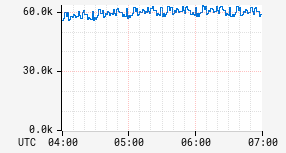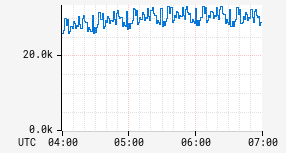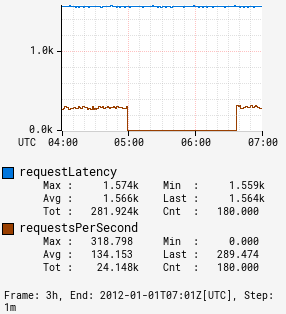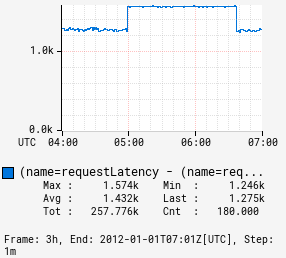# fsub

Input Stack:
 ts2: TimeSeriesExpr ts1: TimeSeriesExpr
Output Stack:
 (ts1 - ts2): TimeSeriesExpr

Floating point subtraction operator. Compute a new time series where each interval has the value `(a - b)` where `a` and `b` are the corresponding intervals in the input time series.

:fsub 1.0 0.0 1.0 NaN NaN
Input 1 2.0 0.0 1.0 1.0 NaN
Input 2 1.0 0.0 0.0 NaN NaN

Note in many cases `NaN` will appear in data, e.g., if a node was brought up and started reporting in the middle of the time window for the graph. This can lead to confusing behavior if added to a line that does have data as the result will be `NaN`. Use the sub operator to treat `NaN` values as zero for combining with other time series.

Example subtracting a constant:

 Before After```name,sps,:eq ``` ```name,sps,:eq, 30000,:fsub ```

Example subtracting two series:

 Before After```name,requestLatency,:eq, :sum, name,requestsPerSecond,:eq, :max, (,name,),:by ``` ```name,requestLatency,:eq, :sum, name,requestsPerSecond,:eq, :max, (,name,),:by, :fsub ```